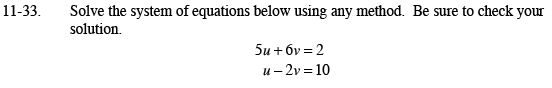### Home > CAAC > Chapter 11 > Lesson 11.1.3 > Problem11-33

11-33.Use either the Substitution or the Elimination method.

Rewrite the second equation in u = form.Then substitute the u = into the first equation.

Multiply the second equation by 3. Then add the equations together.

Solve for both u and v. Substitute your values into both equations to verify that your answer is correct.